I couldn’t find the new tests on the spreadsheet. Could you do SAT test 5, section 4, problem 29 please?

Yeah, I need to add those. Thanks for the reminder!

To get this question, you need to know 1) what the standard circle equation looks like, and 2) how to complete the square. So let’s tackle those in order.

First, here’s the equation of a circle in standard form, in which the circle is centered atand has a radius of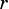:Therefore, what you need to do is take what you’re given and complete the squares to get it into the standard form above. Here’s what that looks like:Recognize thatis the beginning of the binomial squareand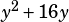is the beginning of the binomial square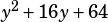. Therefore, we need to add 100 and 64 to both sides of the equation: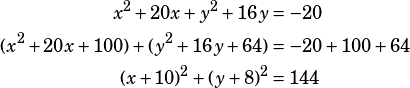From there, you know that the circle’s center is at. (Remember: the standard form has negatives, so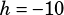in this case.)